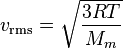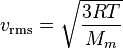Kinetic theory of gases - Maximum speed

If the aleatory speed of a gas rises with pressure and temperature and there is not an apparent limit for these two, what prevents the particles to reach the speed of light?

thank you

Drakkith
Staff Emeritus
Have you ever heard of Relativity? It explains that as you increase the speed of an object it requires more and more energy to increase it further. For example, the Large Hadron Collider accelerates protons up to beyond 99% the speed of light. The energy required to get them from 80% to 99%+ is MORE than it took to get them to 80% in the first place.

Have a look at relativity on wikipedia or do a search for google. Also, I read an interesting book called "The Anime Guide To Relativity" I believe. It explains the basics pretty well. It's a good read if you enjoy anime or you don't like very technical books.

okay, so
Root mean square speed is the measure of the speed of particles in a gas that is most convenient for problem solving within the kinetic theory of gases. It is defined as the square root of the average velocity-squared of the molecules in a gas. It is given by the formulait is saying that, higher the speed of the particles, higher the temperature (or in the other way, idk)

so if it is impossible to particles to reach the speed of light, it is impossible to temperatures get beyond some another value (c².Mm/3.R), or you can't apply this relation to higher speeds?

Drakkith
Staff Emeritus
That equation will not work when you get to speeds that are a significant fraction of the speed of light. You must use relativistic equations at that point, otherwise your numbers become more and more inaccurate the higher you go.
Edit: Just FYI, I don't know the correct formula to use in this situation. I just know that at very high speeds you must use relativistic formulas instead of classical ones.

Redbelly98
Staff Emeritus
Homework Helper
okay, so
Root mean square speed is the measure of the speed of particles in a gas that is most convenient for problem solving within the kinetic theory of gases. It is defined as the square root of the average velocity-squared of the molecules in a gas. It is given by the formulait is saying that, higher the speed of the particles, higher the temperature (or in the other way, idk)

so if it is impossible to particles to reach the speed of light, it is impossible to temperatures get beyond some another value (c².Mm/3.R), or you can't apply this relation to higher speeds?
That formula is based on the assumption that the kinetic energy of a gas molecule is $\frac{1}{2} m v^2$, which is a nonrelativistic approximation. That approximation does not hold at relativistic speeds, and hence the expression $v_{rms} = \sqrt{3RT / M_m}$ is not valid.

thank you, people

First of all, speed of light can't be reached by any means because of energy required is infinity

In addition there is certain limit to the temperature. I've heard there is some "highest temperature that could be reached". But I couldn't remember

And at that enormous velocity it would be no gas any more. Have you seen any gas in stars?

That formula is based on the assumption that the kinetic energy of a gas molecule is $\frac{1}{2} m v^2$, which is a nonrelativistic approximation. That approximation does not hold at relativistic speeds, and hence the expression $v_{rms} = \sqrt{3RT / M_m}$ is not valid.

What about using relativistic mass? I think that Tayloring γ factor would give terms like that

Drakkith
Staff Emeritus
ZealScience, are you saying that there is no gas in stars? If so, that is incorrect. Also, you cannot use relativistic mass for that formula. In fact, i think relativistic mass already gives you the kinetic energy, so there's no need to get the correct answer and try to use it in an inaccurate equation.

ZealScience, are you saying that there is no gas in stars? If so, that is incorrect. Also, you cannot use relativistic mass for that formula. In fact, i think relativistic mass already gives you the kinetic energy, so there's no need to get the correct answer and try to use it in an inaccurate equation.

Which star is made of gas? It must have extremely low temperature comparing to normal stars.

I think taylor the (γ-1)mc^2, there would be a term like that. And if you plug in some moderately high speed, you can still find it a good approximation.

D H
Staff Emeritus
The correct answer is that a relativistic Maxwell-Boltzman gas follows the Maxwell-Juttner distribution, and no, you cannot do a simple gamma correction. The problem is that the Maxwell-Boltzmann distribution has a long tail (excess kurtosis =~ 0.108). The high-speed particles in that long tail are subject to greater relativistic effects than are the particles near the mean.

However, relativistic effects typically are of no concern with regard to thermodynamics. The temperature has to be incredibly high, and the particles incredibly small, for relativistic effects to come into play. For a cloud of electrons, you need to worry about relativistic effects if the temperature is on the order of 1010 K or higher. The last time that such conditions existed was about a second after the big bang.

Drakkith
Staff Emeritus
Which star is made of gas? It must have extremely low temperature comparing to normal stars.

Quote from wikipedia:

The coolest layer of the Sun is a temperature minimum region about 500 km above the photosphere, with a temperature of about 4,100 K. This part of the Sun is cool enough to support simple molecules such as carbon monoxide and water, which can be detected by their absorption spectra.

cjl